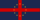5

## Dispatch → Meta → Reference

byThe Villainous Globalist Kittehs of Imperium Anglorum. . 223 reads.

## Data extracts, 14 October 2019Data extracts, 14 October 2019

Hello,

This is a supplement to the increasingly old History of Europe Factbook. I just wanted to give some updates on the current state of Europe's population and endorsement counts. Since measurements of raw endorsements are not available historically, I'll have to use delegate endorsements as a proxy. I have two graphs here.

But first, some explanation about methodology. The words "proportional WA population" refer to the proportion of WA members in Europe relative to the number of WA members in all of the Pacifics. "Yield rate" means the proportion of endorsements given to the Delegate relative to WA members in Europe as a whole.

This is the graph of Proportional WA population and the Yield rate. The blue line is the proportional WA population. Its axis is on the left. The purple line is the yield rate. Its axis is on the right. After a major jump, proportional WA population has remained ready hovering around 8 per cent.

However, we observe a generalised fall in the yield rate over time. As this is our main proxy metric for endorsement counts, this should be of some concern.This is the graph of Delegate endorsements and WA population, from which those figures are based.

Please note a number of things.

From the second graph, it seems obvious that the number of people in Europe since December 2018 has been falling. This is true in raw terms. It is not, however, so in relative terms (that is, the population of the region relative to the populations of the Pacifics). Relative to the site writ large, Europe has not preformed particularly badly.With pleasure, I am yours,
IA

If you are interested in data science, these figures were produced in Python using Matplotlib with the following below.

```import matplotlib.pyplot as plt
import matplotlib.dates as mdates

# visualisation
f, a = plt.subplots(figsize=(297 * 0.0393701 * 0.75, 210 * 0.0393701 * 0.75))

a.plot(pop_data['day'], pop_data['europe_pc_wa'], color='tab:blue')

yield_ax = a.twinx()
yield_ax.plot(pop_data['day'], pop_data['yield_rate'], color='tab:purple')

# axes stuff
a.set_ylabel('WA population (% of total Pacific WA population)', color='tab:blue')
yield_ax.set_ylabel('Yield rate (in %)', color='tab:purple')
a.tick_params(axis='y', labelcolor='tab:blue')
yield_ax.tick_params(axis='y', labelcolor='tab:purple')

a.set_xlabel('Date')
a.xaxis.set_major_formatter(mdates.DateFormatter('%Y-%m'))
a.xaxis.set_major_locator(mdates.MonthLocator(interval=6))
a.xaxis.set_minor_locator(mdates.MonthLocator(interval=1))

a.set_title('Proportional WA population and Yield rate')

f.tight_layout()
f.show()
f.savefig('prop_wa_pop__yield_rate.png')

# visualisation ii
color1 = 'black'
color2 = 'tab:blue'
f, a = plt.subplots(figsize=(297 * 0.0393701 * 0.75, 210 * 0.0393701 * 0.75))

a.plot(pop_data['day'], pop_data['wa_population'], color=color1)

yield_ax = a.twinx()
yield_ax.plot(pop_data['day'], pop_data['delegate_endorsements'], color=color2)

# axes stuff
a.set_ylabel('WA population', color=color1)
yield_ax.set_ylabel('Delegate endorsements', color=color2)
a.tick_params(axis='y', labelcolor=color1)
yield_ax.tick_params(axis='y', labelcolor=color2)

a.set_xlabel('Date')
a.xaxis.set_major_formatter(mdates.DateFormatter('%Y-%m'))
a.xaxis.set_major_locator(mdates.MonthLocator(interval=6))
a.xaxis.set_minor_locator(mdates.MonthLocator(interval=1))

a.set_title('WA population and Delegate endorsements')

f.tight_layout()
f.show()
f.savefig('wa_pop__del_endos.png')```Welcome | Government | Dispatches